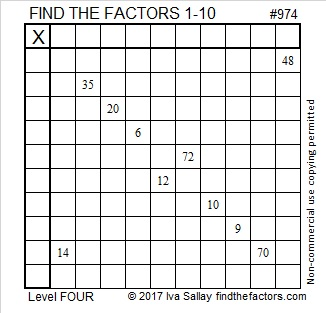# 974 and Level 4

This puzzle has ten clues and, like always, it has only one solution. If you can figure out where to put the factors 1 to 10 in the first column as well as the top row, then you will have found that solution.Print the puzzles or type the solution in this excel file: 10-factors-968-977

This is my 974th post.

974 is the sum of three consecutive square numbers:
17² + 18² + 19² = 974

• 974 is a composite number.
• Prime factorization: 974 = 2 × 487
• The exponents in the prime factorization are 1 and 1. Adding one to each and multiplying we get (1 + 1)(1 + 1) = 2 × 2 = 4. Therefore 974 has exactly 4 factors.
• Factors of 974: 1, 2, 487, 974
• Factor pairs: 974 = 1 × 974 or 2 × 487
• 974 has no square factors that allow its square root to be simplified. √974 ≈ 31.20897